## Linear Independent

In the previous topic of Basis Vector , you have learned that a set of vectors can form a coordinate system in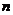dimensional space. The set of vectors that can form a coordinate system is called basis vectors . One main characteristic of basis vectors is that they cannot be put as a linear combination of other basis vector. This characteristic is called linearly independent vectors. Before we discus about linearly independent vectors, we will discuss about linearly dependent vectors.

# Linearly Dependent Vectors

A set of vectors of the samedimensions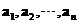is said to be linearly dependent if there is a set of scalars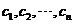, not all zero, such that the linear combination is a zero vector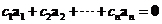.

Linearly dependent vectors cannot be used to make a coordinate system. Geometrically, two vectors are linearly dependent if they point to the same direction or opposite direction. These linearly dependent vectors are parallel or lie on the same line (collinear). Three vectors are linearly dependent if they lie in a common plane passing through the origin (coplanar).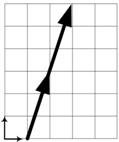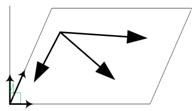Algebraicly, we can augment the set of vectors to form a matrix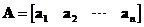sizeby. If the matrix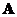is singular (i.e. it has no inverse), then we say that the set of vectorsare linearly dependent.

# Linearly Independent Vectors

Having discussed about linearly dependent vectors, now we are ready for linearly independent vectors.

A set of vectors that is not linearly dependent is called linearly independent. When you put linearly independent vectors in the form of linear combination, the only correct answer would be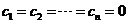because linearly independent vectorscannot be put as a linear combination of one another. Since that is the main characteristics of basis vectors, we say that basis vectors are equivalent to linearly independent vectors.

Geometrically, linear independent vectors form a coordinate system.

By inspection we can determine whether a set of vectors is linearly independent or linearly dependent. If at least one vector can be expresed as a linear combination (i.e. scalar multiple or sum) of the other vectors, then the set of vectors is linearly dependent. If no vector can be expressed as a linear combination of the other vectors, then the set of vectors is linearly independent.

Examples:
A set of vectors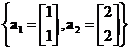is linearly dependent because we can expresed as a scalar multiple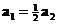.
A set of vectors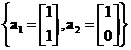is linearly independent because we cannot find a scalar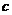such that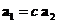.
A set of vectors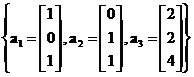is linearly dependent because we can expresed as a linear combination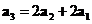.

Algebraicly, the vectorscomprising the columns ofbymatrixsize are linearly independent if and only if the matrixis non-singular (i.e. it has inverse).

For two vectors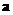and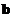, simpler & faster computational procedure in a computer program is based on Cauchy-Schwartz inequality which stated that the absolute value of vector dot product is always less than or equal to the product of their norms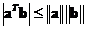. The equality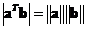holds if and only if the vectors are linearly dependent. Thus, the inequality indicates the vectors are the linearly independent.

The interactive program below is designed to answer whether two vectors are linearly independent or linearly dependent.

vector x vector y